# Precalculus : Matrices and Vectors

## Example Questions

### Example Question #13 : Determine If Two Vectors Are Parallel Or Perpendicular

Which of the following pairs of vectors are parallel?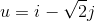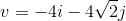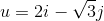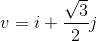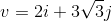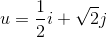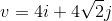Explanation:

For two vectors,andto be parallel,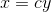, for some real number.

Recall that for a vector,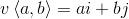.

The correct answer here is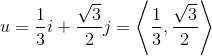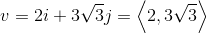.

Using the formulawe have ourto be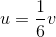.

Applying this we find the vector that is parallel.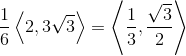### Example Question #11 : Determine If Two Vectors Are Parallel Or Perpendicular

Which pair of vectors represents two parallel vectors?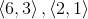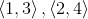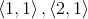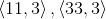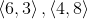Explanation:

Two vectors are parallel if their cross product is. This is the same thing as saying that the matrix consisting of both vectors has determinant zero.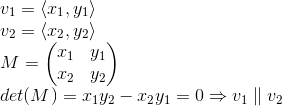This is only true for the correct answer.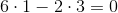In essence each vector is a scalar multiple of the other.

### Example Question #15 : Determine If Two Vectors Are Parallel Or Perpendicular

Which relationship best describes the vectors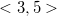and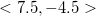?

parallel

neither parallel nor perpendicular

perpendicular

the same direction, but different magnitudes

perpendicular

Explanation:

We can discover that these vectors are perpendicular by finding the dot product: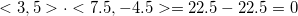A dot product of zero for two non zero vectors means that they are perpedicular vectors.

### Example Question #31 : Parallel And Perpendicular Vectors In Two Dimensions

Which relationship best describes the two vectors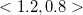and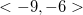?

perpendicular

neither parallel nor perpendicular

parallel

both parallel and perpendicular

parallel

Explanation:

To show that these are parallel, we have to find their magnitudes using the Pythagorean Theorem: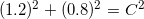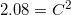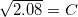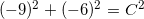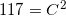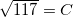To multiply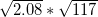, multiply the numbers within the radicals first, then take the square root: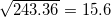Now we have to find the dot product and compare it to the product of these two magnitudes. If they are the same, or if they differ only by sign [one is the negative version of the other] then the two lines are parallel.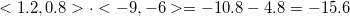This is the negative version of the magnitudes' product! This means that the two vectors are parallel.

### Example Question #1 : Find The Product Of A Matrix And A Scalar

Find the product.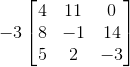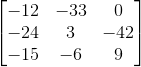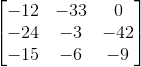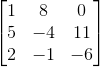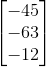Explanation:

When we multiply a scalar (regular number) by a matrix, all we need to do is mulitply it to every entry inside the matrix: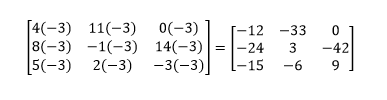### Example Question #2 : Multiplication Of Matrices

Find the product.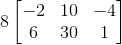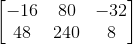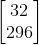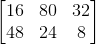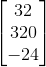Explanation:

When we multiply a scalar (regular number) by a matrix, all we need to do is mulitply it through to every entry inside the matrix: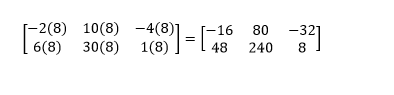### Example Question #3 : Multiplication Of Matrices

Find the product.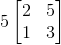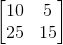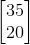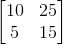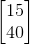Explanation:

When we multiply a scalar (regular number) by a matrix, all we need to do is mulitply it through to every entry inside the matrix: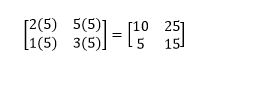### Example Question #4 : Multiplication Of Matrices

We consider the following matrix: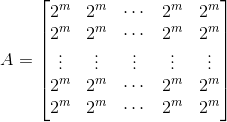let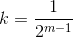what matrix do we get when we perform the following product: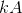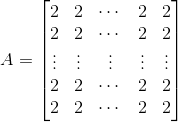The product depends on knowing the value of m.

We can't perform this multiplication.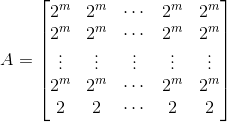The product depends on knowing the size of AExplanation:

We note k is simply a scalar. To do this multiplication all we need to do is to multply each entry of the matrix by k.

we see that when we multiply we have :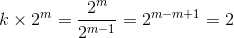this gives the entry of the matrix kA.

Therefore the resulting matrix is :### Example Question #5 : Multiplication Of Matrices

We consider the matrix defined below.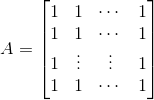Find the sum :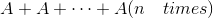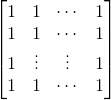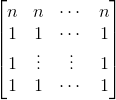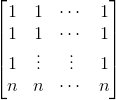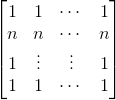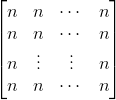Explanation:

Since we are adding the matrix to itself, we have the same size, we can perform the matrices addition.

We know that when adding matrices, we add them componenwise. Let (i,j) be any entry of the addition matrix. We add the entry form A to the entry from B which is the same as A. This means that to add A+A  we simply add each entry of A to itself.

Since the entries from A are the same and given by 1 and the entries from B=A are the same and given by 1, we add these two to obtain:

1+1 and this means that each entry of A+A is 2. We continue in this fashion by additing the entries of A each one to itself n times to obtain that the entries of A+A+....A( n  times ) are given by:### Example Question #6 : Multiplication Of Matrices

Letbe a positive integer and letbe defined as below: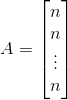Find the product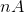.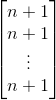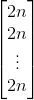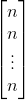We can't multiplyand.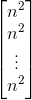Explanation:

We note n is simply a scalar. To do this multiplication all we need to do is to multply each entry of the matrix by n.

We see that when we multiply we have :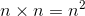.

This means that each entry of the resulting matrix is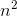.

This gives the nA which is :### All Precalculus Resources